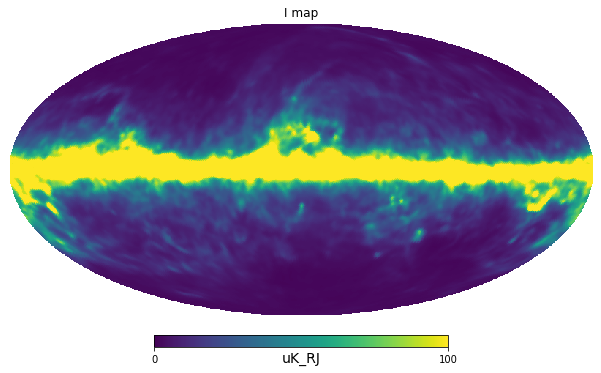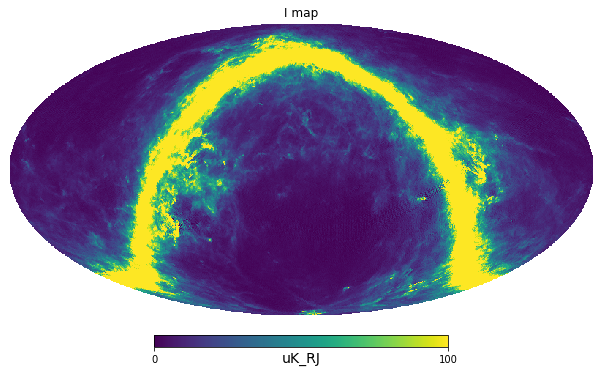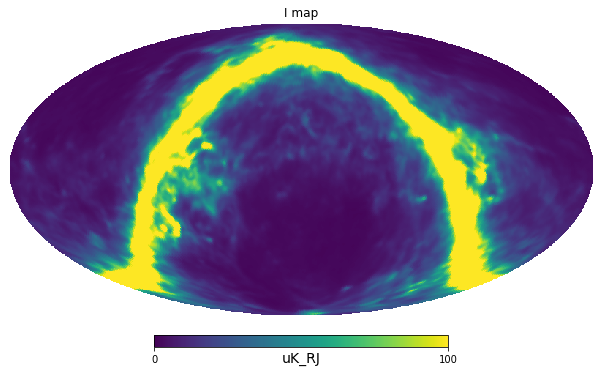# Smoothing and coordinate transformation¶

Smoothing and coordinate transforms are combined because they are both performed in spherical harmonics space, so we can have a single transform to and from $$a_{lm}$$.

Currently they are implemented as an indepent function pysm3.apply_smoothing_and_coord_transform which takes an input map and returns another map either smoothed or coordinate-transformed or both. Usually it operates on maps created with pysm3.Sky.get_emission but it would work even with other inputs.

:

sky = pysm3.Sky(nside=128, preset_strings=["d1", "s1"])

:

map_100GHz = sky.get_emission(100 * u.GHz)

:

def plot_map(m):
"""Utility function to plot Mollweide view of a map"""
hp.mollview(m, min=0, max=1e2, title="I map", unit=m.unit)

:

map_100GHz_smoothed = pysm3.apply_smoothing_and_coord_transform(map_100GHz, fwhm=2*u.deg)
plot_map(map_100GHz_smoothed):

map_100GHz_rotated = pysm3.apply_smoothing_and_coord_transform(map_100GHz, rot=hp.Rotator(coord="GE"))
plot_map(map_100GHz_rotated):

map_100GHz_smoothed_rotated = pysm3.apply_smoothing_and_coord_transform(map_100GHz, rot=hp.Rotator(coord="GE"), fwhm=2*u.deg)
plot_map(map_100GHz_smoothed_rotated)[ ]:



[ ]: